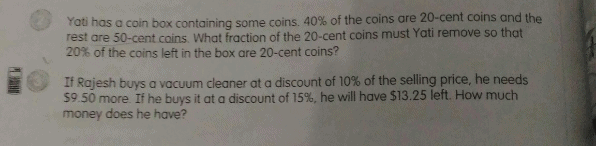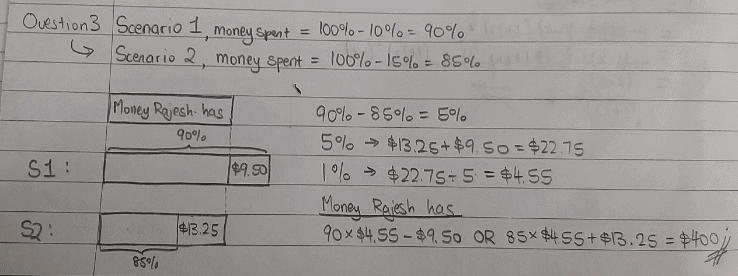# QuestionHi…can anyone assist me for question number 2 and 3. Thanks advance..That’s for Question Three. Hope you understand!

0 Replies 1 Like

QUESTION TWO

20-cent coins, before = 40u

20-cent coins, after = 20p

50-cent coins (unchanged) = 100u – 40u = 60u and 100p – 20p = 80p

60u = 80p

3u = 4p

So, if you convert p into u,

20-cent coins, after = (20p ÷ 4p) x 3u = 15u

So, 20-cent coins, removed is 40u – 15u = 25u

Fraction of 20-cent coins removed is 25/40 = 5/8 (simplified)

Hope you understand and all the best for your exams! :))

0 Replies 1 Like